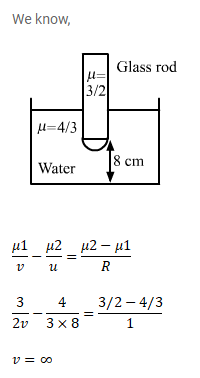# One end of a cylindrical glass rodQuestion:

One end of a cylindrical glass rod $(\mu=1.5)$ of radius $1.0 \mathrm{~cm}$ is rounded in the shape of a hemisphere. The rod is immersed in water $(\mu=4 / 3)$ and an object is placed in the water along the axis of the rod at a distance of $8.0 \mathrm{~cm}$ from the rounded edge. Locate the image of the object.

Solution: